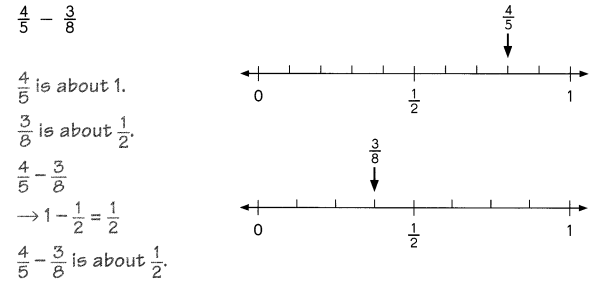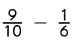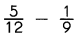# Math in Focus Grade 5 Chapter 3 Practice 2 Answer Key Subtracting Unlike Fractions

Go through the Math in Focus Grade 5 Workbook Answer Key Chapter 3 Practice 2 Subtracting Unlike Fractions to finish your assignments.

## Math in Focus Grade 5 Chapter 3 Practice 2 Answer Key Subtracting Unlike Fractions

Rewrite the fractions as like fractions and complete the subtraction sentence.

Example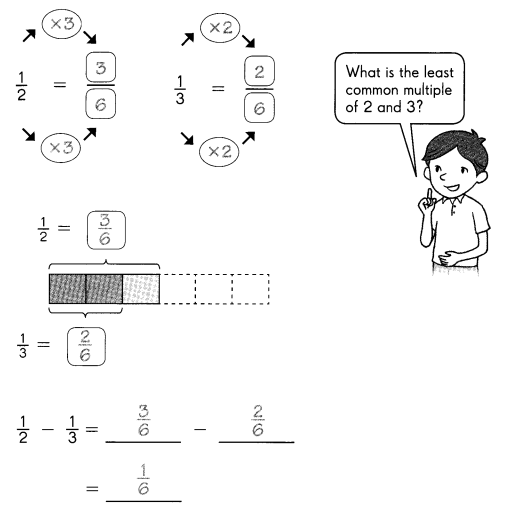Rewrite the fractions as like fractions and complete the subtraction sentence.

Question 1.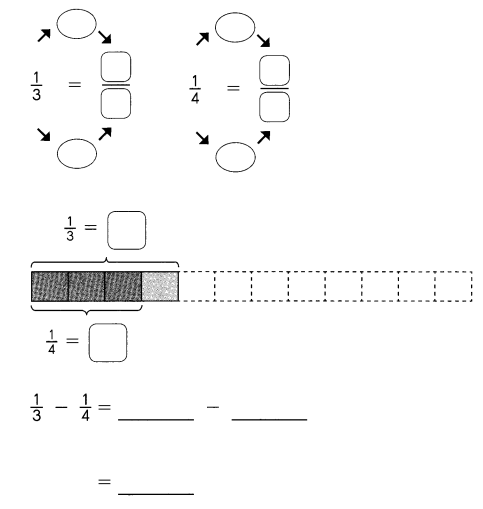Subtract. Express each difference in simplest form.

Question 2.
$$\frac{7}{12}$$ – $$\frac{2}{4}$$ =

Question 3.
$$\frac{4}{5}$$ – $$\frac{1}{3}$$ =

Question 4.
1 – $$\frac{5}{6}$$ – $$\frac{1}{12}$$ =

Question 5.
$$\frac{7}{9}$$ – $$\frac{1}{6}$$ =